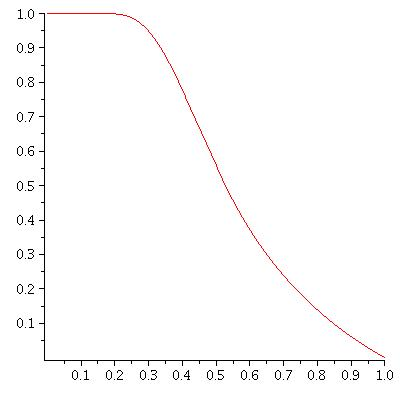The Histogram of the Fractional Counting of Integer partitions of 2000The horizontal axis is k/n (for n=2000). The vertical axis is the fractional count of partitions of n whose largest part is exactly k (where each partition is given a "weight" equal to the reciprocal of the product of its parts)

Added Nov. 14, 2018: Here is a plot of Christopher Ryba's function described in his article. We thank Christopher Ryba for permission to reproduce it.(Notice the similarity!)

back to article's front-page